# Solid Figures and Volume

There are two types of three-dimensional figures that are covered in the official scope of the GMAT:

• Rectangular solids, otherwise known as rectangular prisms; and
• Cylinders.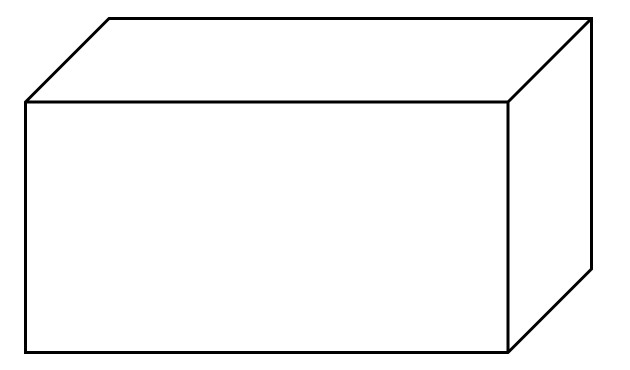A rectangular solid

When dealing with a rectangular prism, you may need to compute the area or the surface area. If the dimensions of the solid are a, b, and c, then the area is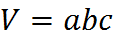and the surface area is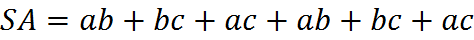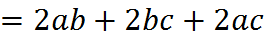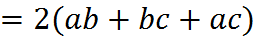When calculating the surface area, we are simply calculating and adding the area of 3 pairs of rectangles. If you think of the dimensions as length, width, and height, then the (same) equation is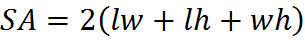A convenient way to think of the volume equation is as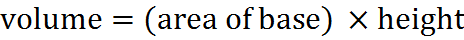In the case of a rectangular solid, the “base” could be any one of the sides, comprising two dimensions, and the “height” is the length of the shape’s extension along the final dimension.

This area for volume applies not only to rectangular solids, but also to cylinders: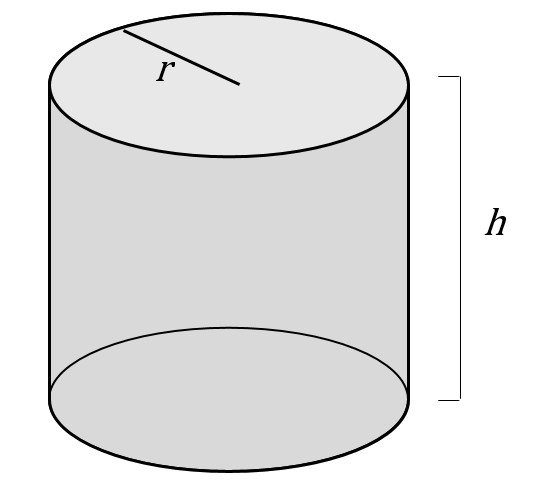In this case, the base is a circle. If we are told that the cylinder is a “right regular cylinder,” or otherwise we know that the height dimension is perpendicular to the base, then we obtain the volume simply by multiplying the area of the circle times the height: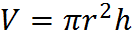The surface area of this cylinder consists of the top circle, the bottom circle, and the single curved side. If you think of the single curved side as a label or wrapping on the cylinder, you can imagine unwrapping it and see that it’s a rectangle of height h and a length equal to the circumference of the circle. Therefore, the surface area is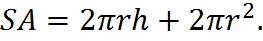Finally, believe it or not, the volume of a sphere is not mentioned within the official scope of GMAT math, but to appease the skeptics among you I will remind you that the formula for a sphere’s volume is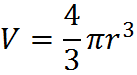## Practice Questions

Volume of Two Boxes:
http://www.gmatfree.com/volume-of-two-boxes

Distance within a Box:
http://www.gmatfree.com/distance-within-a-box

Volume from Area:
http://www.gmatfree.com/volume-from-area

Welcome! You are encouraged to register with the site and login (for free). When you register, you support the site and your question history is saved.